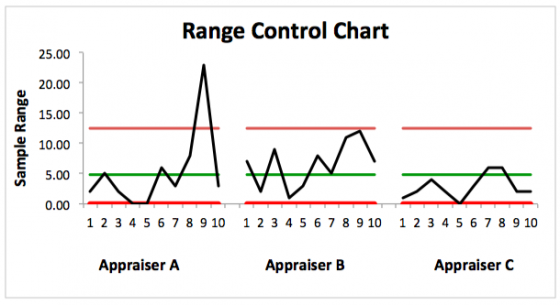# Statistical Process Control

Statistical Process Control (SPC) charts provide warning signs when processes exhibit unusual behavior.  This can be valuable in (1) detecting special-cause variation before too many defective products are produced and (2) gaining a better understanding of the process and reducing  unwanted variation.  The downside of control charts (the exception is pre-control) is that they normally do not coincide with specification limits, so a process that is acting normally according to control chart rules can still produce parts that are out of specification if the process is not capable to begin with.

Here is an explanation of the most common types of control charts and when they are used –

## Statistical Process Control for Variable Data

Variable data is measured and plotted on a continuous scale.  Examples include measurements taken with micrometers or calipers.  Here are the most common variable-data control charts –

1.  X-Bar and R Chart – Subgroup Size of 2 to 9 Samples

The X-Bar and R Chart is the most commonly used variable-data control chart, and is used when the subgroup sample size (the number of parts pulled and measured at each inspection) is in the two to nine range.  A subgroup sample size of five is very typical.  Two charts make up the X-Bar and R chart:  one plots the subgroup average and the other plots the subgroup range.  Each chart has upper and lower control limits to detect out-of-control conditions.  These charts are also very useful in reviewing Gage R&R results.  Here is an R-Chart from a Gage R&R study that shows an out-of-control data point with Appraiser A on  Part 9.  Understanding the reason for the this out-of-control data point can lead to improved results:2.  Individuals and Moving Range – Subgroup Size of 1

The Individuals and Moving Range chart is used when a single part is measured at each inspection time.

3.  Average and Standard Deviation Chart – Subgroup Sizes Greater Than 9

Also known as the “X-Bar and S” chart,  this chart is used for very high subgroup sizes, and is much less common than chart types (1) and (2) above.

4.  Median and Range Chart – Subgroup Sample Sizes in the 3 to 5 Range

Median and Range charts are rarely used, and are considered an alternative to X-Bar and R charts when the sample average cannot easily be calculated due to workforce or shop floor limitations.

## Statistical Process Control for Attribute Data

Attribute control charts can focus on one feature at a time like variable control charts, but can also expand to include defect counts and percent defective.  Here are the most common types of attribute control charts –

1. Fraction Defective (p Chart) – Subgroup Sizes Typically Greater than 50, Variable Subgroup Samples Acceptable

p charts track the percentage of nonconforming units in a large sample size to help the team know when a given defect rate or pattern is statistically abnormal.  p charts can be used for tracking data over long periods of time, such as shifts and weeks.  These charts can also work with variable subgroup sizes since the fraction defective is plotted on the chart.

2. Number Defective (np Chart) – Constant Subgroup Size, Typically 50 or More

np charts monitor the total defective unit quantity when a constant subgroup size is used.

3.  Number of Defects (c Chart) – Constant Subgroup Size

While p and np charts monitor defective units (a defective unit is one that contains one or more defects), c and u charts monitor total defects, so they are useful when multiple potential defects exist for each part.

4.  Defects Per Units (u Chart) – Variable Sample Size

A u chart monitors the average defects per unit, which is very useful when variable sample sizes (such as units built per shift) are involved.

## Constructing and Interpreting Control Charts

There are a number of guidelines available for constructing and interpreting control charts.  Here is a good set of guidelines.